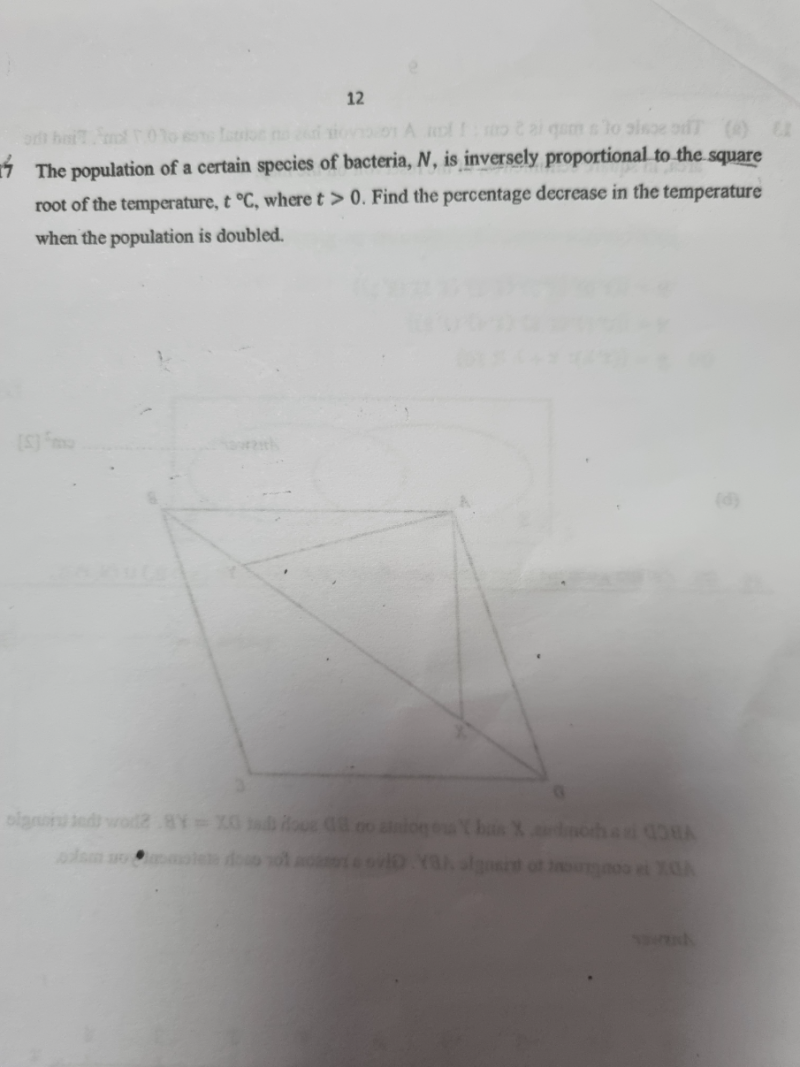# QuestionThank you

N = k/ sqrt(t)  where t > 0 and k = constant

N2  = k2 / t

=> t = (k/N)2

old temperature = (k /N )2  = k2 / N2

new temperature = (k/2N)2  = k2 / 4N2    (when N is doubled)

Δt =  new temperature – old temperature

=   (   k2 / 4N2 –  k2 / N2

=   ( k – 4k2 ) / 4N2

= – 3/4  (k/N)2

Since it is negative, it is a reduction and converting to % = 3/4 x 100% = 75%

0 Replies 1 Like